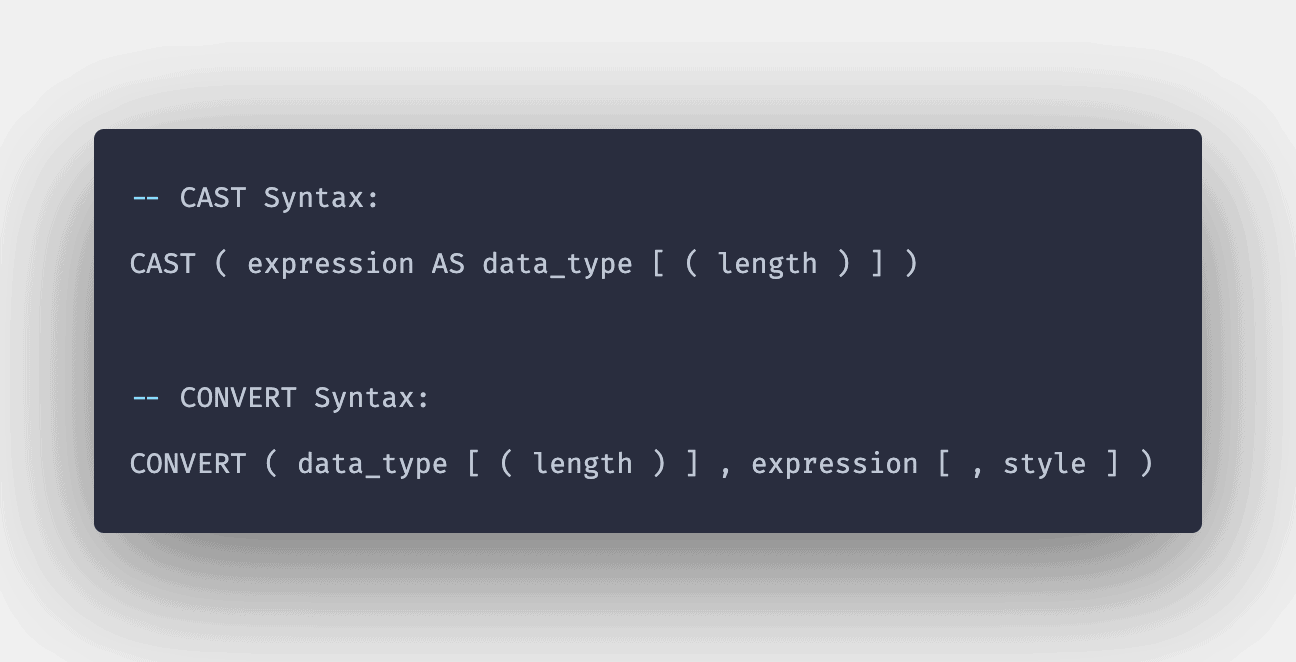# SQL CAST() Function: Complete Guide

0
4The CAST() function converts a value (of any type) into a specified datatype.

## SQL CAST

SQL CAST() is a built-in function that is used for converting an expression from one data type to another data type. If the conversion takes place, a value with a specified conversion will be returned. Otherwise, the function will return an error.

### Syntax

```CAST (expression AS type [ (length)])
```

### Parameters

1. Expression: The value to be converted to another datatype.
2. Type: The data type to which the expression will be converted.
3. Length: It is entirely optional. It signifies the length of the resulting data type for expression.

#### Note

1. The result is truncated when an expression is converted to integer datatype when converted from float or integer.
2. For other conversions, the value is rounded.

### Examples

```SELECT CAST (10.85 AS int);
```

```10
```

#### Explanation

As the expression is converted from float to integer. So, the result here is truncated.

### Query 2

```SELECT CAST (10.85 AS float);
```

```10.85
```

#### Explanation

As the expression is converted to float datatype. So, the result here is not truncated, and the original expression was returned, which was already in the float.

### Query 3

```SELECT CAST (15.6 AS varchar);
```

`'15.6'`

#### Explanation

Here, the floating expression was converted to character datatype.

### Query 4

```SELECT CAST ('15.6' AS float);
```

```15.6
```

#### Explanation

Here, the character data type is converted to a floating-point value.

### Query 5

```SELECT CAST (5.95 AS DEC (3,0));
```

`6`

#### Explanation

Here, the decimal expression is converted to another decimal expression with different lengths.

### Query 6

```SELECT CAST ('2020-02-26' AS datetime);
```

#### Output

```'2020-02-26 00:00:00.000'
```

#### Explanation

Here, the string expression is converted to datetime expression.